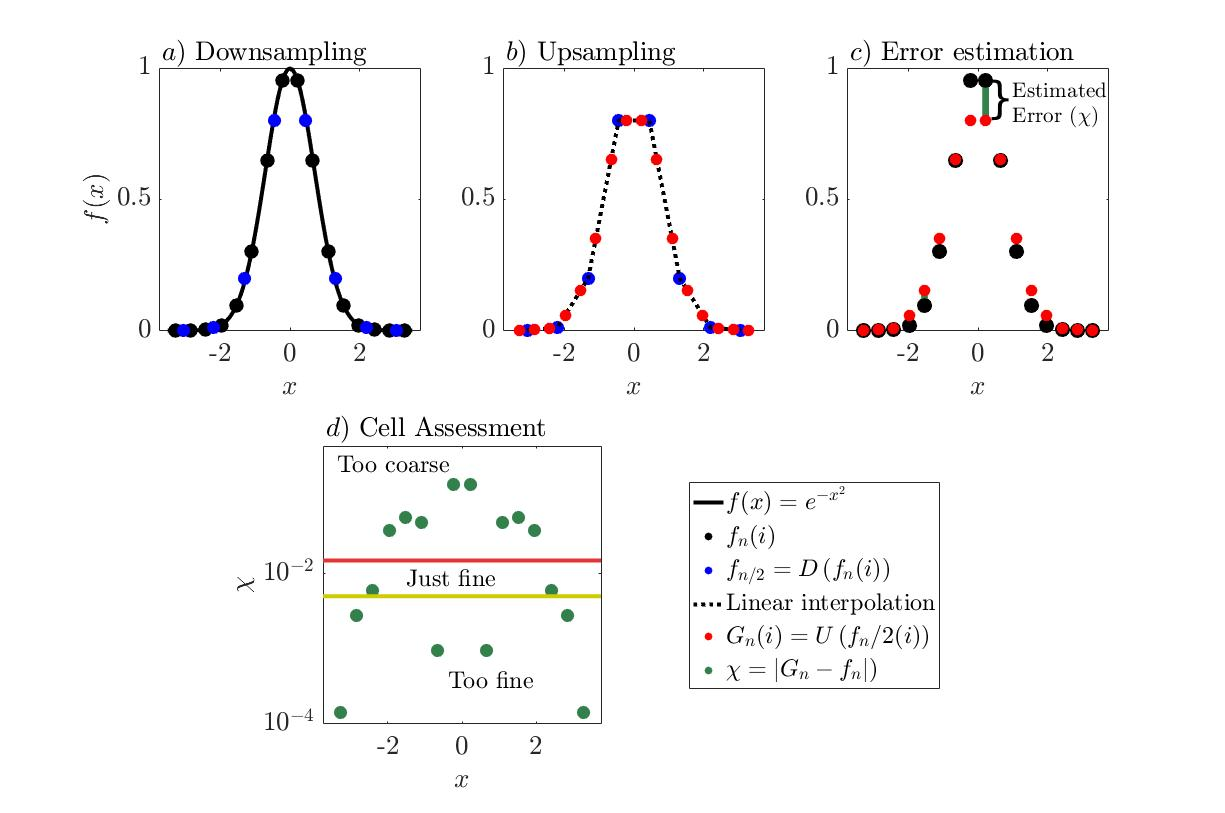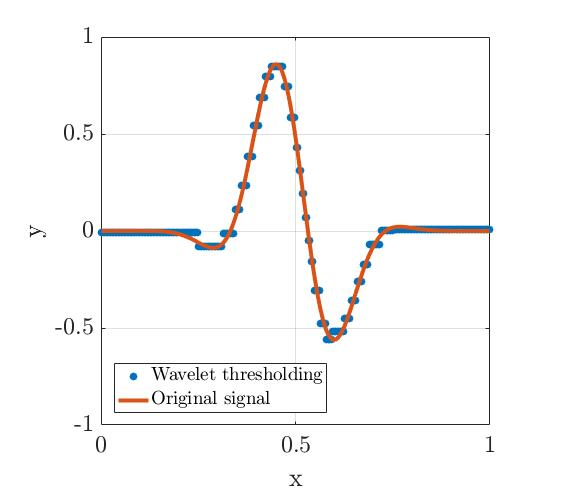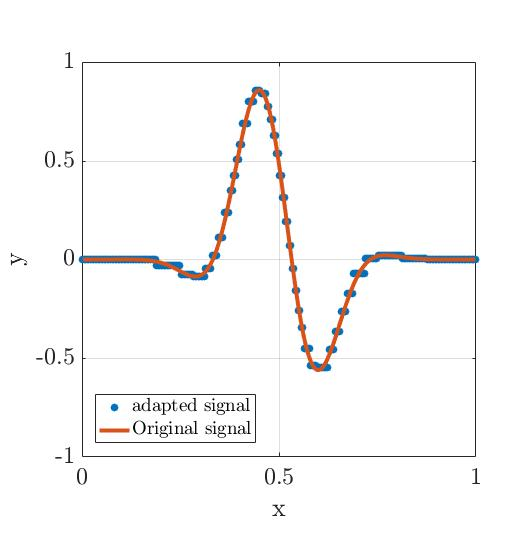# The Grid Adaptation Algorithm Based on a Wavelet-Estimated Discretization Error

Basilisk can employ anisotropic meshes using tree-based grids. If you are not familiar with this grid structure, this link might be helpfull. The tree structure facilitates a convienient basis for refinement and coarsening, such that the mesh resolution can vary over the course of the simulation based on the evolution of the solution itself (i.e. adaptive). This requires a decision algorithm. Ideally such algorithm is generally applicable for a multitude of solvers. Basilisk’s wavelet-based strategy is designed to be such an adaptation algorithm. This page aims to discribe how it can be employed and what goes on behind the scenes. The algorithm is designed and implemented by Stéphane Popinet. It is consisely described in Popinet (2015)

This page was written to guide the writing for another publication, by Van Hooft et al.(2018). You may find a another version of the content that is presented on this page via the link.

## Syntax

The function adapt_wavelet is defined in grid/tree-common.h. In short, the adaptive wavelet algorithm is based arround the estimation of numerical errors in the representation of spatially-discretized fields. The adapt_wavelet() function requires the user to define a list of fields that it will analyze for the refinement/coarsening. This list can consist of any combination of the existing scalar fields. In general, it makes sense to use the fields that appear in the equations that are being solved. Additionally the maximum tolerated estimated error for each field needs to be defined. Finally, a maximum level (i.e. resolution) that the algorithm is allowed to employ should be provided. Optionally, two more inputs can be defined. i.e. 1: the minimum level of refinement and 2: A list of scalars that need to be updated. The default values of these optional arguments are 1 and all, respectively. Adaptation can be done according to the following example event, where two dimensional scalar fields A, B, C and vector field u are present:

...
....

In this example, adaption is done every timestep and is based on the three fields associated with A and u (i.e. u.x & u.y), The maximum tolerance of the estimated error is 0.1, 0.2 and 0.3 for A, u.x and u.y, respectively. The grid is allowed to vary between 3 and 8 levels of refinement. Scalar field C is not updated after adaption, meaning that this field will not have sensible values initialized at locations where the grid is either refined or coarsened. This could be for example a diagnostic field that does not appear in any computation or output routine before its values are computed again.

## Wavelet based error Estimation

The mathematical rigor of the used algorithm is rooted in the field of Multi-resolution analysis. Consider a one dimensional signal f that is discretized using an even number (n) elements, we can write f_n. The i-th entry of the signal f_n is denoted as f^i_n. We now define a down sampling operator (D) that coarsens the original signal to a lower level solution such that,

\displaystyle f_{n/2}=D\left(f_n\right).

Alternatively, we define an upsampling operator (U) such that,

\displaystyle g_{n}=U(f_{n/2}).

Note that in general, g^i_n \neq f^i_n and that the difference defined as,

\displaystyle \chi^i_n = |f^i_n-g^i_n|,

can be interpreted as an error associated with the subsequent application of the downsampling and upsampling operators to the signal f_n. For the downsamping operation we choose local volume-avereaging of the fine resolution solution to obtain a coarse resolution solution. In (Basilisk) practice this means that for a N-dimensional grid, 2^N leaf cells that share a common parent cell are averaged and the resulting value is assigned to the corresponding parent cell. Notice that this operation is exact for a finite volume formulation. For the upscaling operator however, a second order accurate interpolatiton seems suitable as Basilisk (typically) employs second-order-accurate formulations for its solvers. This entails using the just, bi or tri linear interpolation techniques for 1,2 and 3 dimensional fields, respectively, using the coarse level solution. Given the implementation of this formulation one can evaluate \chi(i) and destinguish tree cases regaring the grid cell’ resolution by defining a threshold on the allowed error (\zeta):

\displaystyle \text{The\ }i\text{-th Grid Cell is\ } \begin{cases} &\text{Too Coarse.} & \chi^i_n > \zeta,\\ &\mathrm{Too\ Fine.} & \chi^i_n < \frac{2\zeta}{3},\\ &\mathrm{Just\ Fine.} & \mathrm{Otherwise}, \end{cases}

\zeta represents the so-called refinement criterion that has the same units as f. Notice that since the down and up sampling operator are defined using only local data, the signal f is not required to be defined on an globally equidistant grid. However to ensure a local 3^N coarse grid stencil for the linear interpolation, the resolution between cells is only allowed to vary one level of refinement. The figure below demonstrates how the discribed algorithm accesses the resolution of a signal (f_n) according to a refinement criterium \zeta.A visual representation of the various steps done by the algorithm to assess the resolution of a given grid.

### An example application

This section aims to exemplify how the adaption algorithm assesses a discretized signal and adapts the grid according to a refinement criterion ζ. For this purpose, we apply the algorithm to a subset of the data from the simulation of forced isotropic turbulence in Li et al. (2008). The simulation is run using a fixed equidistant grid with 1024^3 nodes; in terms of the Kolmogorov length scale (η), the grid spacing (\Delta_i) is \Delta_i=2.2η. For the analysis we assume the data to be resolved well enough, and the results are kindly made available via the Johns Hopkins turbulence databases. We analyze a 2D slice of the data (i.e. 1024^2 cells) and for simplicity, we only consider the velocity component perpendicular to the sliced plane (u_⊥). The data are presented in Fig. 5a (see below); using the algorithm described in the previous section, we can evaluate the χ field corresponding to the original u_⊥ field. A section of the resulting field, indicated by the black box in Fig. 5a, is shown in Fig. 5b, where we can clearly see that the estimated discretization error is not distributed uniformly by the equidistant-grid approach that was used in the simulation. Rather, it appears that there are anisotropic structures present, visualized by relatively high χ values (in yellow). These structures appear to correspond to vortex filaments that characterize the dissipative structures of high-Reynolds-number turbulence (Frisch 1995). This result motivates the application of the grid refinement algorithm to the data sample shown. Note that we cannot add new information by refinement and at this point we do not make any claims regarding what χ values are reasonable for a turbulence-resolving simulation (this will depend on the numerical). As such, we only allow the algorithm to coarsen the field with a maximum error threshold ζ. The number of grid cells resulting from the application of the adaptation algorithm for a range of ζ values is shown in Fig. 5c; as expected, the number of grid cells decreases with an increasing ζ value. Note that the plot also shows that even for the high ζ values, the grid still contains cells at the maximum resolution.

The main concept of employing the described grid-adaption algorithm is visualized in Fig.5d. Here histograms of the number of grid cells within 512 equally-spaced χ bins are presented for the original data and the data obtained from applying the grid adaptation technique with three different refinement criteria. It appears that for the original dataset, the histogram is monotonically decreasing with increasing χ. This shows that many grid cells exist where the numerical solution is relatively smooth compared to cells in the tail of the histogram. Hence, if the grid is chosen such that the discretization errors in the latter region do not affect the relevant statistics of the flow evolution, then the grid must be over-refined elsewhere. The histograms of the adapted grids show that the algorithm is able to lower the number of grid cells with low χ values, such that fewer grid cells are employed. Note that the grid coarsening does not introduce new grid cells with χ>2ζ/3, as this part of the histogram remains unaltered.

When grid cells with a small but finite χ value are coarsened, some of the data are lost and in general cannot be exactly reconstructed by interpolation techniques. In order to assess how the data from the adapted grids compare with the original data, Fig. 5e presents the corresponding power spectra. It appears that none of the adapted grid data are able to exactly reproduce the original power spectrum; more specifically, with increasing ζ values, the wavenumbers (k) that show a significant deviation in E(k) from the original appear to decrease. We point out that in order to evaluate the spectrum we have linearly interpolated the data from the non-uniform grids to an equidistant grid with 1024×1024 data points. The choice of the interpolation technique is arbitrary and will pollute the diagnosed spectrum in a non-trivial manner. As such, we directly compare all 10242 u_⊥(x,y) samples in Fig. 5f, where we see that the deviation of the data from the 1 : 1 line is a function of ζ.Fig. 5 Example of the adaption algorithm applied to a 2D slice of a 3D turbulent field. a Shows the data slice of the velocity component in the plane-perpendicular direction (u_⊥, obtained from Li et al. (2008). b Presents the χ field, evaluated using the method described in the previous section Only the centre part of the slice, indicated by the black box in a, is shown to reveal the small-scale details in this simulation. c shows the grid-cell-number dependence on the chosen refinement criterion (ζ), note the logarithmic vertical axis. A histogram of the χ field with 512 bins for the original data, and the data corresponding to three ζ values are presented in d. Using the same colour coding as in d, power spectra and a direct comparison of the u_⊥(y,z) field are shown in e, f, respectively

### The down and upsampling in Basilisk

In Basilisk various different downsampling operations are used. In the code this is referred to as the restriction operation. It is defined as an attribute of a given scalar field. By default basilisk uses the following definition when initializing a new scalar field s (see here):

s.restriction = restriction_average;

The upsampling operation is referred to as prolongation. By default, for a scalar field, this is defined as (see here):

s.prolongation = refine_bilinear;

One may change these attributes if the argumentation for the chosen restriction and prolongation formulation does not apply for your solver formulation. This is for example the case for a volume of fluid (fraction) field c (see here).

c.prolongation = fraction_refine;

Many attributes for prolongation and restriction are defined in the common multigrid header file, but you are free to employ your own definitions, e.g. here.

### So where are the wavelets?

Similar to the beter known Fourier transformation, a wavelet based transformation entails the decomposition of a signal into a set of orthorgonal functions. The main difference is that the used orthogonal functions (\psi) are localized in both physical space (x) as in the frequency domain (f). A wavelet function (\psi(x)) can be rescaled with a factor a (dyadic dilation), and shifted in space by b (dyadic positioning) to obtain a set of wavelet functions,

\displaystyle \psi_{ab}(x)=\psi \left(\frac{x-b}{a}\right),

that may be orthogonal for a prober choice of \psi(x),a and b. Once such choice results in the Haar-wavelets:

\displaystyle \psi_H(x)=\begin{cases} 1 \quad & 0 \leq t < \frac{1}{2},\\ -1 & \frac{1}{2} \leq t < 1,\\ 0 &\mathrm{otherwise.} \end{cases}

Supplemented with a pair of integers n,k that determine the dyadic scaling and positioning, respectively, one obains an orthogonal set of wavelets,

\displaystyle \psi_{nk}=2^{n/2}\psi_H(2^nx-k)

The associated wavelet transform can be carried out discretely using the so-called Haar-transform matrix H for a (1D), discretized signal f_n, \displaystyle W_n=Hf_n,

where W_n is a vector of the coefficients for the various wavelet-components. The idea is that not all components contribute equally to the original signal, so one may choose to do without the components that are smaller than some threshold \zeta,

\displaystyle T_i = \begin{cases} W_i, & |W_i| \geq \zeta,\\ 0, & |W_i| < \zeta. \end{cases}

Here T_i is the results of the so-called wavelet thresholding processes of W_n. We can obtain an approximation of the orginal signal f_n, F_n, \displaystyle F_n = H^{-1}T_n. Let us look the result of such a transformation:Haar transform of a signal containing 256 points using \zeta=0.1

We see that at the locations of low variablility (i.e. low derivative) the signal is approxmimated coarsly, and vise versa.

…SOME TEXT THAT MAKES A CONNECTION…

Let us look what the wavelet-based algorithm of basilisk produces for the same signal, using the following code:

#include "grid/bitree.h"
#include "utils.h"

scalar f[];
int main(){
FILE * fp1 = fopen("original","w");
f.prolongation=refine_injection;
X0=0;
L0=1;
init_grid(256);
foreach(){
f[]= sin(((x)*M_PI*5)-11.5)* exp(-(sq((x-0.5)/0.15)));
fprintf(fp1,"%g\t%g\n",x,f[]);
}
foreach()
f[]= sin(((x)*M_PI*5)-11.5)* exp(-(sq((x-0.5)/0.15)));
boundary({f});
}
double xp=L0/512;
while(xp<L0){
Point point = locate(xp);
fprintf(fp2,"%g\t%g\n",xp,f[]);
xp+= L0/256.;
}
}

We obtain very similar behaviour:Wavelet thresholding using Basilisk

The differences are mostly due to the fact that the Basilisk grid structure only allows resolution boundaries that differ by a factor of two.

## Refinement and coarsening

When the error is estimated for all grid cells they are marked to be either too fine, too coarse or just fine. All cells that are marked to be too coarse will be refined, also cells that require refinement in order to keep the levels at resolution boundaries differ a single level are refined. Coarsening is only done when all children of a single parent cell are marked to be too fine and coarsening those cells does not violate the mentioned requirement.

The techniques used for defing field values on the newly initialized grid cells are not always identical to the restriction and prolongation operation. For this purpose all fields defined on a tree grid also have a refine and coarsen attributes to facilitate distiction between the methods used for prolongation and restriction. The default for scalar fields is (as found in the common tree-grid header file):

...
s.refine = s.prolongation;
...

Furthermore, by default for a scalar field, the coarsening attribute is not even defined and the restriction operation is used instead when cells are coarsened.

## More flexible wavelet-based algorithms

For no particular reason I dare to specify here, there may be situations where the model user is somehow alloted with a priori knowledge on the physical system and its resolution requirements. Such knowledge may be exploited in a way of employing the described algorithm is a more flexible manner. Here we list and link to three notable examples:

The adapt_wavelet() function in combination with: